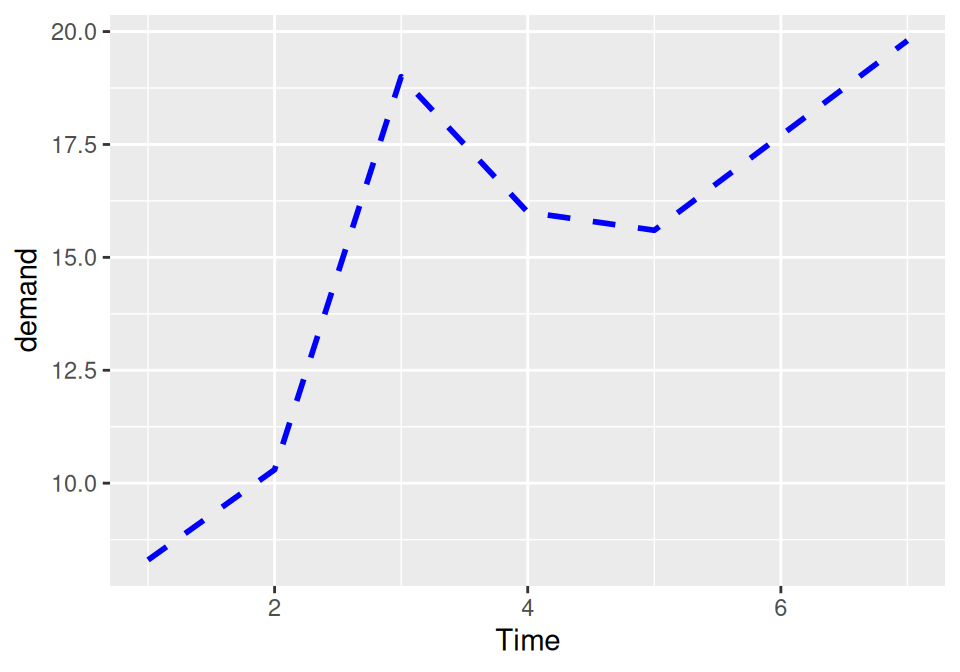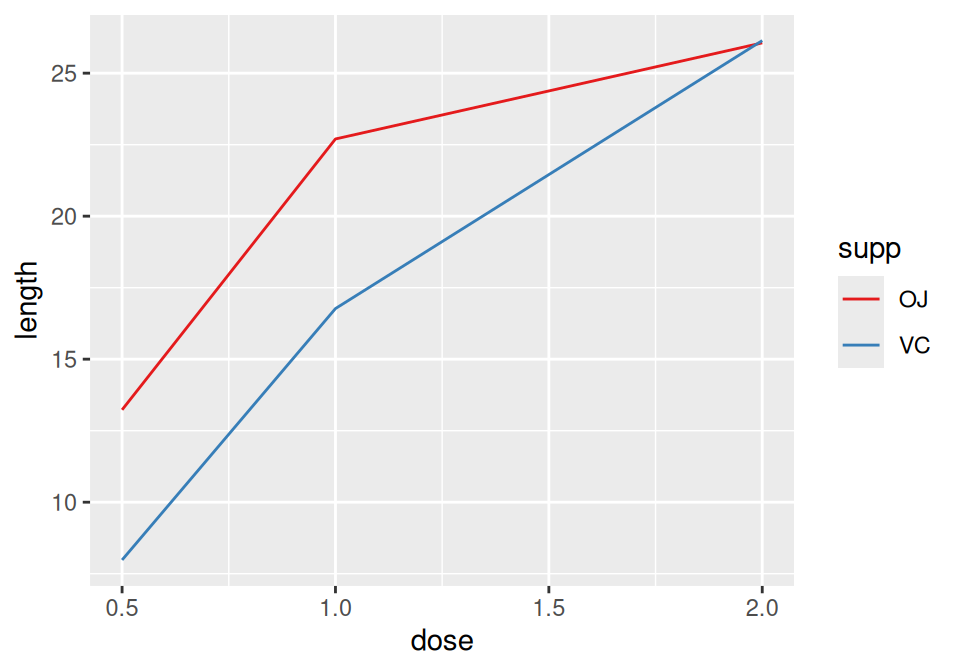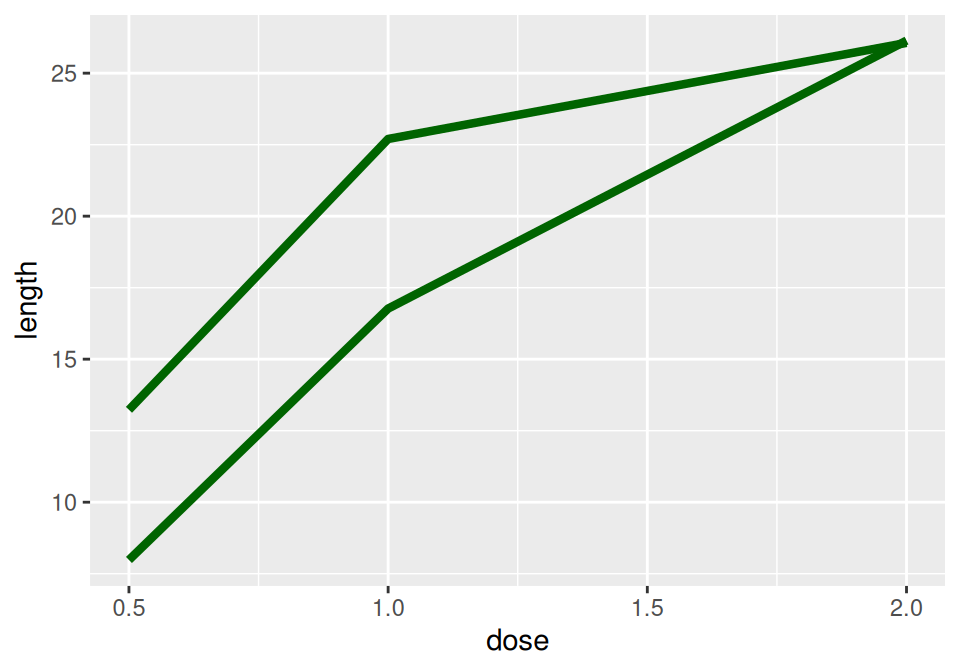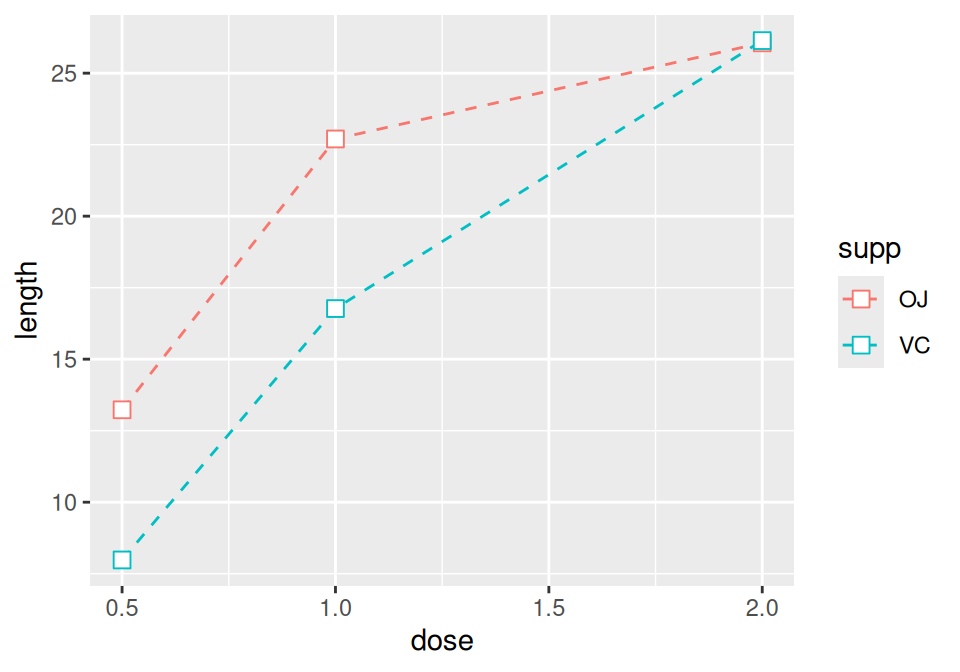## 4.4 Changing the Appearance of Lines

### 4.4.1 Problem

You want to change the appearance of the lines in a line graph.

### 4.4.2 Solution

The type of line (solid, dashed, dotted, etc.) is set with `linetype`, the thickness (in mm) with `size`, and the color of the line with `colour` (or `color`).

These properties can be set (as shown in Figure 4.11) by passing them values in the call to `geom_line()`:

``````ggplot(BOD, aes(x = Time, y = demand)) +
geom_line(linetype = "dashed", size = 1, colour = "blue")``````Figure 4.11: Line graph with custom linetype, size, and colour

If there is more than one line, setting the aesthetic properties will affect all of the lines. On the other hand, mapping variables to the properties, as we saw in Recipe 4.3, will result in each line looking different. The default colors aren’t the most appealing, so you may want to use a different palette, as shown in Figure 4.12, by using `scale_colour_brewer()` or `scale_colour_manual()`:

``````library(gcookbook)  # Load gcookbook for the tg data set

ggplot(tg, aes(x = dose, y = length, colour = supp)) +
geom_line() +
scale_colour_brewer(palette = "Set1")``````Figure 4.12: Using a palette from RColorBrewer

### 4.4.3 Discussion

To set a single constant color for all the lines, specify colour outside of `aes()`. The same works for size, linetype, and point shape (Figure 4.13). You may also have to specify the grouping variable:

``````# If both lines have the same properties, you need to specify a variable to
# use for grouping
ggplot(tg, aes(x = dose, y = length, group = supp)) +
geom_line(colour = "darkgreen", size = 1.5)

# Since supp is mapped to colour, it will automatically be used for grouping
ggplot(tg, aes(x = dose, y = length, colour = supp)) +
geom_line(linetype = "dashed") +
geom_point(shape = 22, size = 3, fill = "white")``````Figure 4.13: Line graph with constant size and color (left); With `supp` mapped to `colour`, and with points added (right)